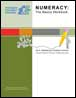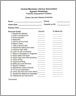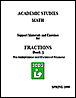# Division

## Numeracy: The Basics Workbook – Set L: Dividing Decimals (2012)This is one in a series of workbooks designed to accompany Workplace Education Manitoba’s (WEM’s) Numeracy: The Basics video series. The series contains 50 videos, covering 25 numeracy topics.

The workbook is divided into five sections, with problems getting more complex as learners progress. Solutions for all the problems can be found at the back of the workbook.

2013-07-26

## Numeracy: The Basics Workbook – Set E: Multiplying & Dividing Fractions (2012)This is one in a series of workbooks designed to accompany Workplace Education Manitoba’s (WEM’s) Numeracy: The Basics video series. The series contains 50 videos, covering 25 numeracy topics.

This workbook is divided into five practice sections that focus on multiplying and dividing fractions. Solutions for all the problems are provided in the workbook.

2013-07-10

## Dividing Decimals - Practice [Video – 8:46] (2011)With this video tutorial, learners get the chance to practise the skills required to divide decimals.

The tutorial includes three division problems, each a little more complicated than the one before. Learners can pause the video while they work out the problems on their own, then restart it to see the instructor’s detailed solutions to the problems.

2012-05-11

## Dividing Decimals - Concept [Video – 19:52] (2011)This video introduces the concepts underlying the process of dividing with decimals.

The instructor narrating the video explains that division with decimals requires the use of long division, with special attention to the position of the decimal point.

He leads the learner through a series of problems, solving each one in a series of steps and offering tips to simplify the calculations.

2012-05-11

## Multiplying & Dividing Fractions – Practice [Video – 8:33] (2011)This video tutorial gives learners a chance to practise multiplying and dividing with fractions.

The tutorial includes three problems. Learners can pause the video while they work out the problems on their own, then restart it to see the instructor’s detailed solutions.

2012-03-20

## Multiplying & Dividing Fractions – Concept [Video – 14:30] (2011)This math video tutorial introduces the principles for multiplying and dividing with fractions.

The instructor points out that multiplication and division of fractions, unlike addition and subtraction, do not require a common denominator.

He uses several problems to illustrate the two operations and also explains how to reduce fractions to their simplest form at various stages of the calculations.

2012-03-20

## Deeper Into Math (2005)Deeper into Math is a math workbook focuse on multiplication, division, fractions and decimals. Each section consists of an explanation of the concept followed by practical exercises. Before tackling the concepts in this workbook, students should complete the pretest included in the book to make sure they have the necessary addition and subtraction skills.

2008-12-15

## Portfolio Assessment Initiative (2001)This portfolio assessment initiative has several lists of goals for students and teachers. It includes:
- personal goals
- reading progress checklist
- listening and speaking progress checklist
- writing progress checklist and,
- mathematics progress checklist

2006-08-10

## Academic Studies Math - Fractions: Part 3 - The Multiplication and Division of Fractions (1999)

Support Materials and ExercisesThis manual deals with the multiplication and division of fractions. It also includes support materials and exercises.

2005-11-01

## The Next Step: Mathematics Applications for Adults - Book 14018 (2003)The Book 14018 section of the "Next Step" manual for adults demonstrates the mathematics applications for Number Operations; Fractions, Decimals, and Percent; Percent, Ratio, and Proportion; Geometry; Measurement, Integers; Equations: Equalities and Inequalities; Graphs.